Next: Conclusion and future work Up: A Review of Monte Previous: Bi-directional Path Tracing

# Bi-directional Estimators for Light Transport

Veach proposed a bi-directional ray-tracing algorithm that is similar to the previous one, but it uses combined sampling techniques [VG94]. In this algorithm the eye path and the light path are only connected at the end points by a deterministic shadow ray. The pixel intensities are estimated according to the Monte Carlo method: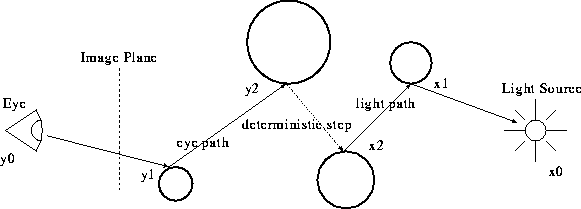Figure: A complete transport path
```ESTIMATE-PIXEL(P)

sum=0
for n=1 to N
for k=1 to Max-length
x=CHOOSE-PATH(P,k)
sum=sum+f(x)/p(x)
return sum/N

```

CHOOSE-PATH(P,k) generates a path x of length k which potentially contributes to the intensity of pixel P. f(x) is the differential contribution to P of light flowing along x,and p(x) is the probability density with which CHOOSE-PATH generates x.

Veach proposed combining sampling techniques for generating random paths, where the distribution of the samples is constructed from several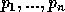importance sampling distributions. The relative number of samples taken from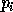is denoted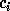, where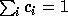. Thevalues are fixed in advance before any samples are taken. The resulting distribution can be written as: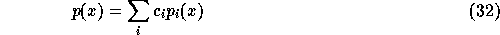.

Using the combining sampling techniques unbiased and low variance estimators can be constructed that allow samples to be weighted differently, depending on which distributionthey where chosen from. Each estimator is parametrised by a set of weighting functions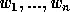, where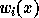gives the weight associated with a sample x drawn from. The combined estimator is given by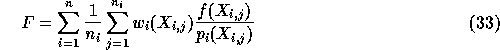where the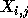is the jth sample from distribution. For the unbiased estimation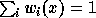for all x.

Csébfalvi Balázs
Tue Apr 15 18:39:13 METDST 1997